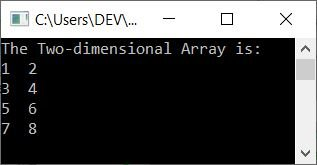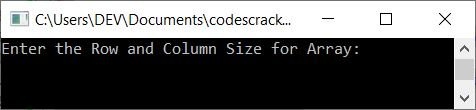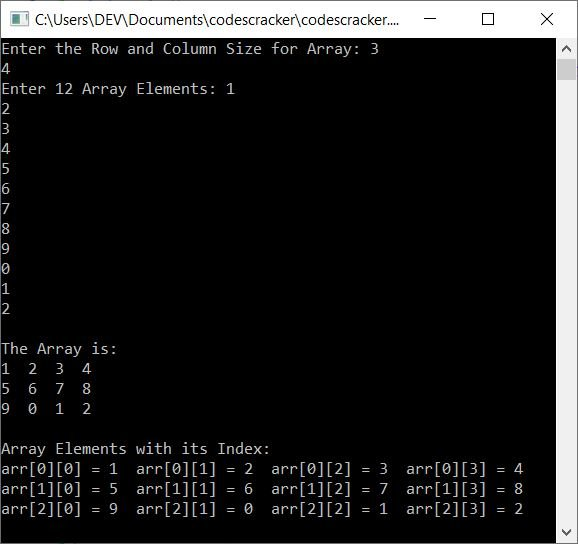# C++ Program for Two-Dimensional (2D) Array

In this article, you will learn and get code to implement a two-dimensional (2D) array in C++. Here is the list of programs on the 2D array:

Note: A two-dimensional (2D) array can be thought of as a matrix with rows and columns. For example:

```1 2 3
4 5 6```

is a two-dimensional array with two rows and three columns in size.

## Two-Dimensional Array Program in C++

This program initializes 8 elements in a two-dimensional array of size four rows and two columns, then prints the array on output:

```#include<iostream>
using namespace std;
int main()
{
int arr = {{1, 2}, {3, 4}, {5, 6}, {7, 8}};
int i, j;
cout<<"The Two-dimensional Array is:\n";
for(i=0;  i<4; i++)
{
for(j=0; j<2; j++)
cout<<arr[i][j]<<"  ";
cout<<endl;
}
cout<<endl;
return 0;
}```

This program was built and runs under the Code::Blocks IDE. Here is its sample output:Note: The outer for loop is responsible for rows, and the inner for loop is responsible for columns.

## Get Array Elements of the Given Size from the User

Now this program allows the user to enter the dimension or size of a 2D array and then its elements of the given size to store it in a 2D array arr[][] and print the array back on the output screen along with the index number (row and column number starting from 0):

```#include<iostream>
using namespace std;
int main()
{
int row, col, i, j, arr;
cout<<"Enter the Row and Column Size for Array: ";
cin>>row>>col;
cout<<"Enter "<<row*col<<" Array Elements: ";
for(i=0; i<row; i++)
{
for(j=0; j<col; j++)
cin>>arr[i][j];
}
cout<<"\nThe Array is:\n";
for(i=0; i<row; i++)
{
for(j=0; j<col; j++)
cout<<arr[i][j]<<"  ";
cout<<endl;
}
cout<<"\nArray Elements with its Index:\n";
for(i=0; i<row; i++)
{
for(j=0; j<col; j++)
cout<<"arr["<<i<<"]["<<j<<"] = "<<arr[i][j]<<"  ";
cout<<endl;
}
cout<<endl;
return 0;
}```

The snapshot given below shows the initial output produced by this program:Now supply inputs of 3 as the row size and 4 as the column size of the array, then 1, 2, 3, 4, 5, 6, 7, 8, 9, 0, 1, 2 as the 12 elements for the array:#### The same program in different languages

C++ Quiz

« Previous Program Next Program »